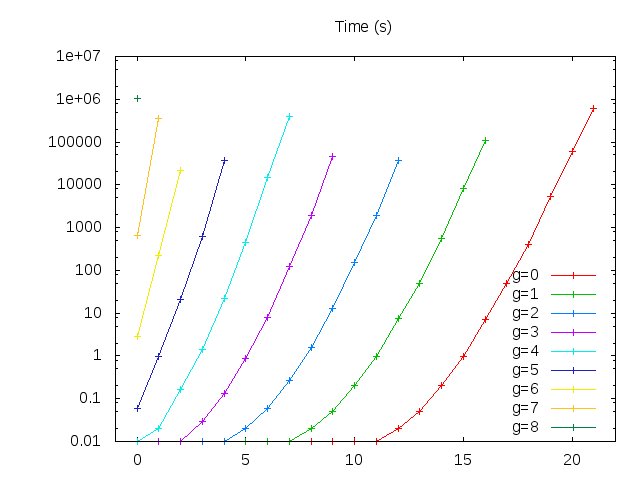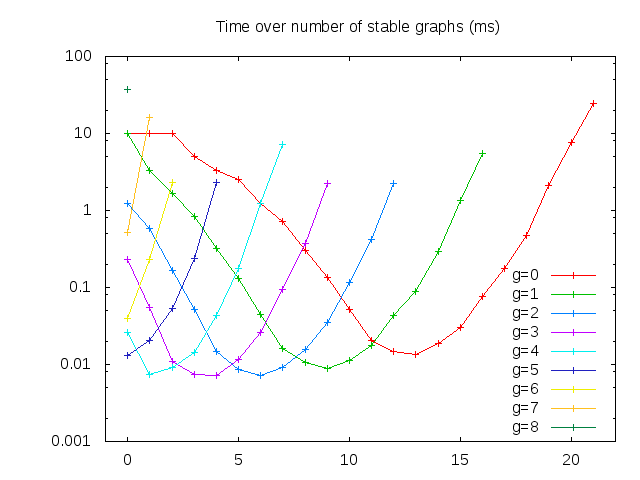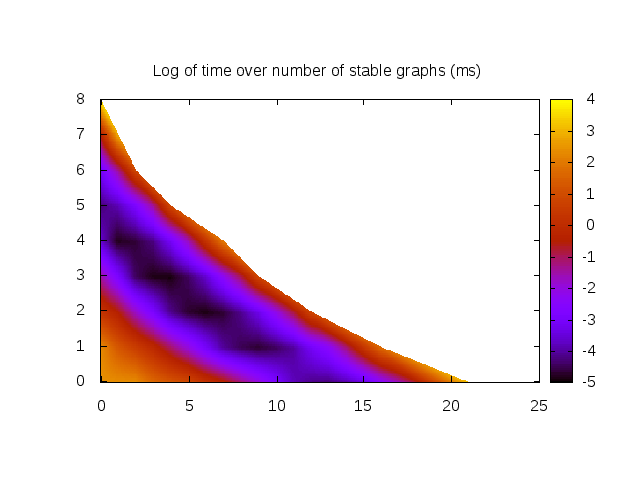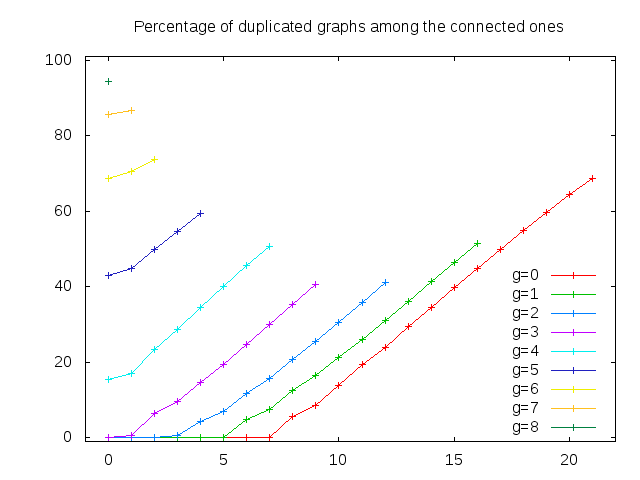# Boundary graph computer for the moduli space of stable pointed curves

### Authors: Stefano Maggiolo & Nicola Pagani

Given two natural numbers g and n satisfying 2g+n-2>0, the program generates all genus g stable graphs with n marked points. Each such graph determines the topological type of a nodal stable curve of arithmetic genus g with n marked points. Our motivation comes from the fact that the boundary of the moduli space of stable genus g, n-pointed curves can be stratified by taking loci of curves of a fixed topological type.

You can also download the current version of the paper and the program at our public repository.

To compile the program, first you have to download the latest version of nauty, extract it inside boundary/nauty. Then do `./configure && make all` inside nauty's directory, and finally `make` inside boundary's directory.

## Output

The program can print stable graphs in several ways. For small values of g and n, the most useful should be the LaTeX print, which prints a stand-alone LaTeX source that you can compile (if you have PGF/TikZ installed) to obtain something like this PDF that contains all stable graphs with g = 3 and n = 1.

Otherwise, the handier output format is binary format (enable it with the switch `-P B` on the command line). The format is described in details at the beginning of the source file `graph.h` and it is designed to be as compact as possible. We already computed the binary output for some dozens of cases (the one plotted in the following); if you need them you can send an email to one of the authors.

## Some benchmarks

Number of stable graphs for fixed g, when n varies:Time (s) for fixed g, when n varies:Time over number of stable graphs (s) for fixed g, when n varies:Time (s) for n = 0:Time (s) for g = 0:Heat map of log of time (ms):Heat map of log of time (ms) over # of stable graphs:Heat map of log of time (ms) over # of graphs generated (stable and unstable):Heat map of log of # of stable graphs:Heat map of the percentage of unconnected graphs amongst all the graphs generated:Heat map of the percentage of duplicated graphs amongst the connected graphs generated:Percentage of duplicated graphs amongst the connected graphs generated: